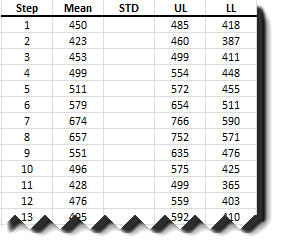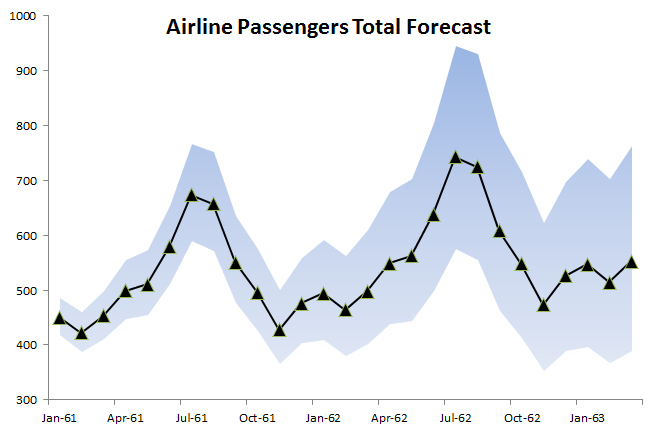# Text Book Example - Airline Passenger Data

In this paper, we will analyze the international passenger data series (G), as mentioned in the textbook Time Series: Forecast and Control by Box, Jenkins, and Reinsel (ISBN: 978-0470272848). This textbook was first published in the late 1960s and is considered by many practitioners as the definite foundation textbook on the time series topic.

The international airline passenger series describes monthly totals of the international passengers for the period between Jan 1949 and Dec 1960.

The objective here is to follow the same analysis in the book and to demonstrate the accuracy of NumXL calculations. Furthermore, SAS – a leading statistical software vendor – demonstrate their own analysis for the same data set, so we strongly recommend our users to review their results as well, via this link:

SAS Procedural reference - Example 7.2 Seasonal Model for the Airline Series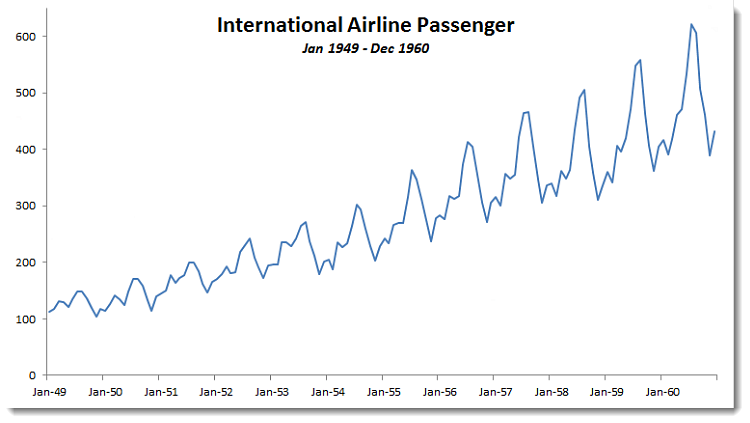## Step 1: Data Transformation

Using the Descriptive Statistic wizard (pictured below), examine the different summary stats of the sample data.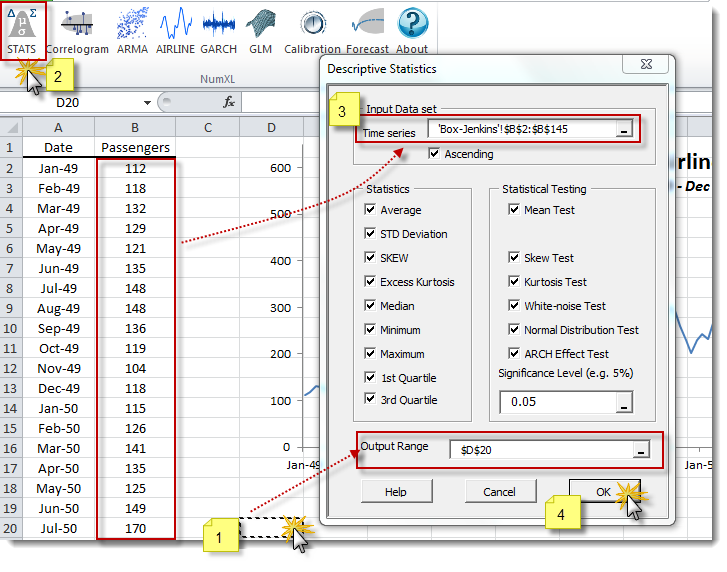In the summary statistics table (pictured below), the data series exhibits serial correlation (i.e. failed the white noise test) and fat tails (significant excess kurtosis and ARCH effect).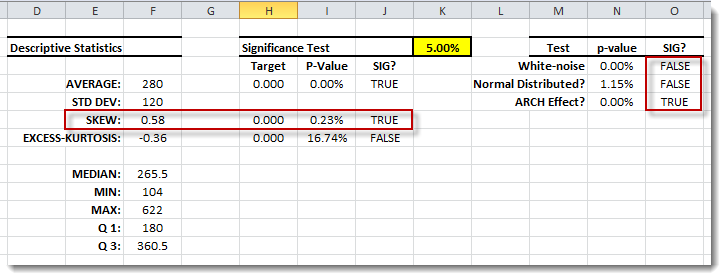The original analysis converts the data series using the natural logarithm function (i.e. LN). Follow the same technique, as shown in the graph below: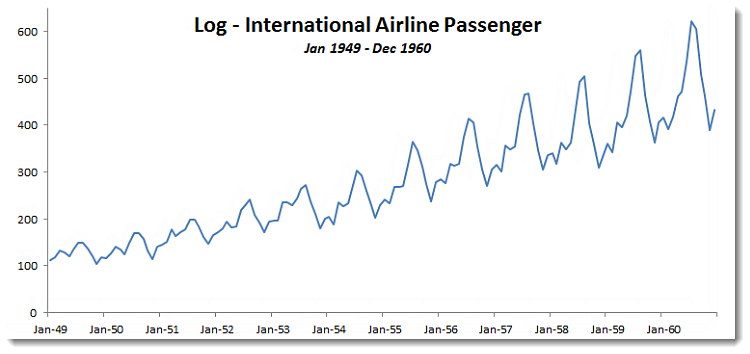This technique should yield the following summary statistics: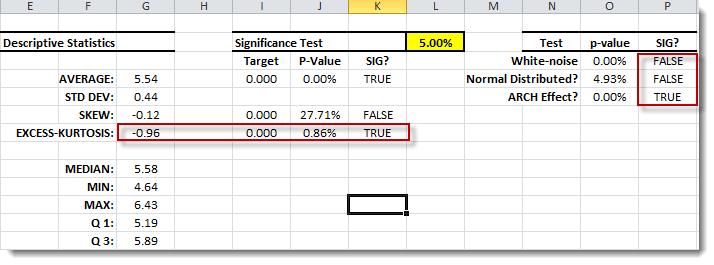Notice that the transformed data series is smoother than the original data and the time trend appears more linear than the original.

## Step 2: Correlogram Analysis

Using the NumXL toolbar, launch the Correlogram wizard.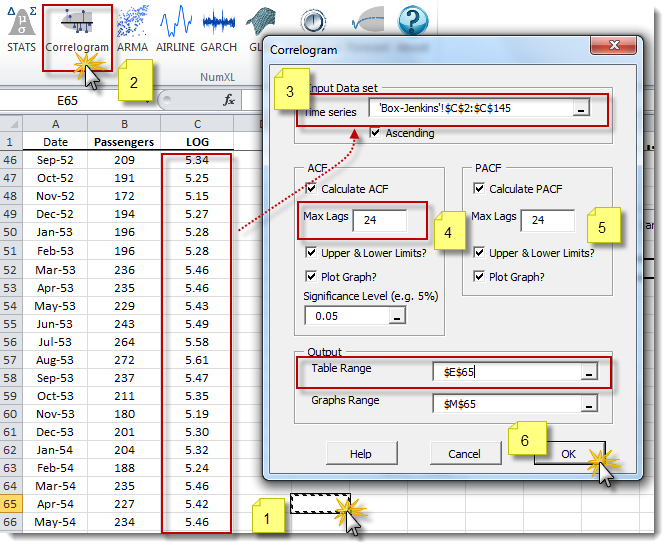Highlight the log data and select 24 lags for ACF and PACF. Then, create a correlogram for the data.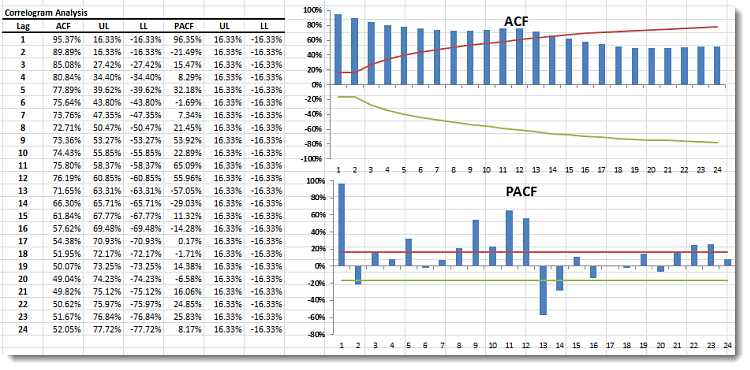Examining the ACF plot, the data appear to be integrated at lag one(1) and at lag twelve (12). Difference the data for both lags (i.e. $\left(1-L\right)\left(1-L^{12}\right)$),as shown in the graph below: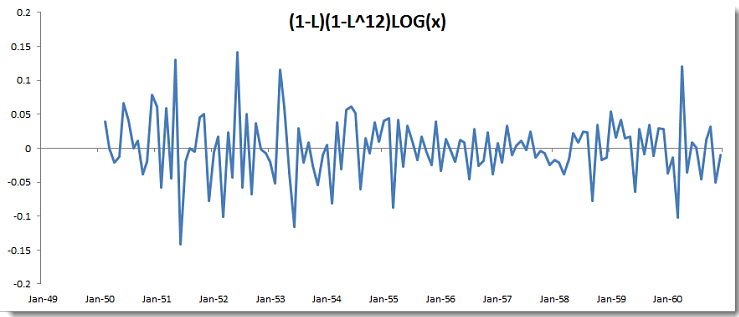The differenced data set should generate the following correlogram: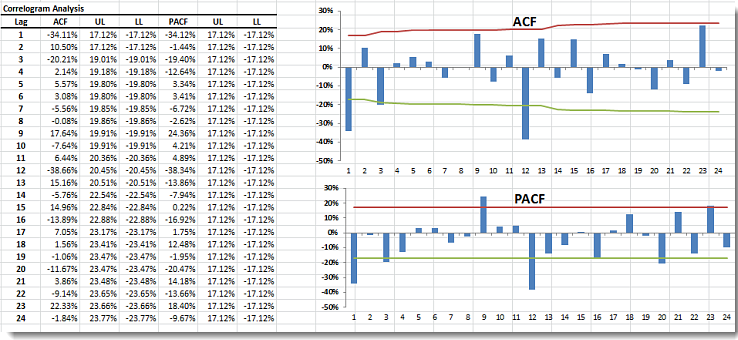Also, notice that the ACF plot of the differenced data series shows a significant autocorrelation at lag one (1) and lag twelve (12).

## Step 3: Airline Modeling

The proposed model for the log passenger data series is an airline model with a season length of 12 months.

$$\left(1-L\right)\left(1-l^{12}\right)\ln{X_t}=\mu \left(1+\theta L\right)\left(1+\Theta L^{12}\right)a_t$$

Where

• L = the backshift operator (aka B).
• $a_t$ = the error term, shock, innovation, or simply the model residual at time t.
• $\mu$ = the mean of the seasonal differenced time series.

Using the NumXL toolbar, click on the Airline icon to launch the Airline model wizard.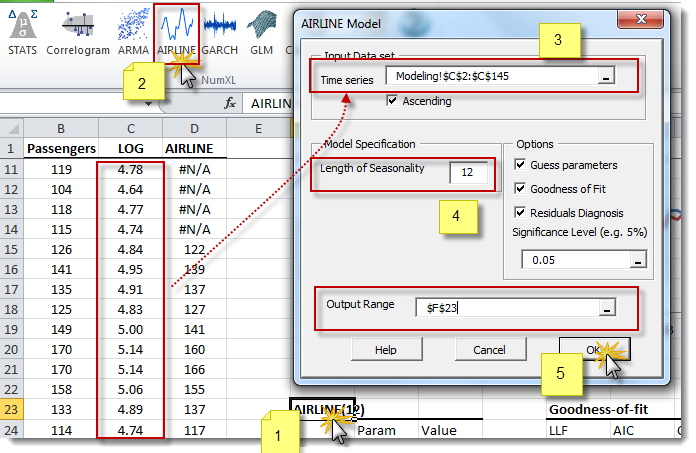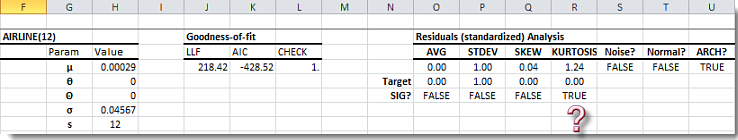## Step 4: Calibration

Select the cell at the top of the airline model table (i.e. “AIRLINE(12)”) and click on the Calibration icon in the toolbar.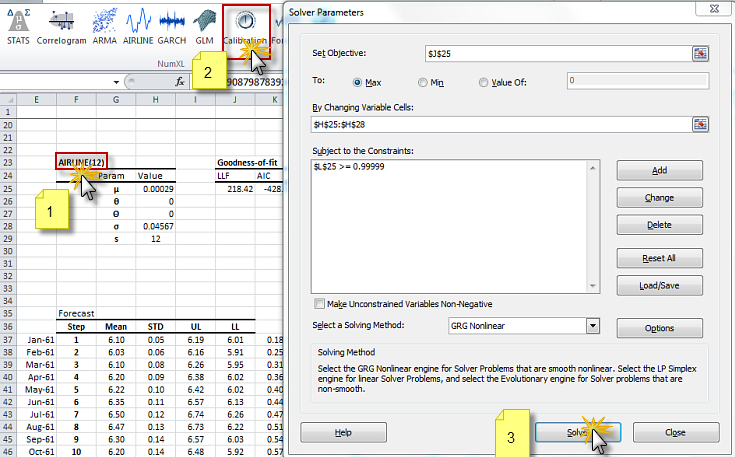The Excel solver will try to determine the optimal values for the airline model’s parameters (i.e. $\theta,\Theta$ )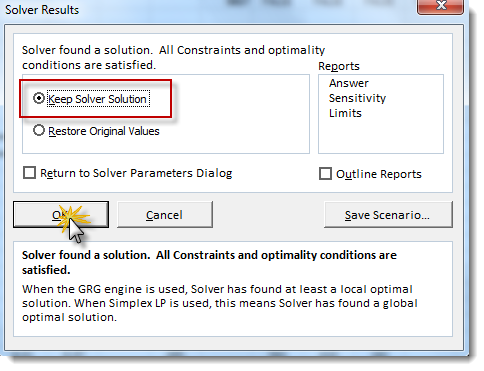The new optimal values for the model parameters are shown below: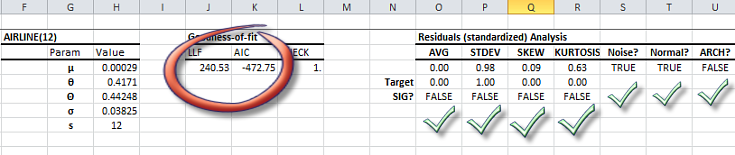Examining the residuals analysis table, the calibrated values satisfy all assumptions of the underlying model (i.e. Gaussian distributed residuals).

The parameter values of the calibrated model on the SAS website are slightly different from the ones we calculated earlier: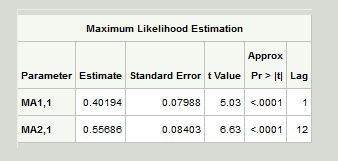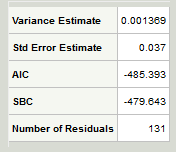However, our values are within the error tolerance limits (i.e. ) and our Akaike’s information criterion (AIC) is better.

The core difference between NumXL and SAS values - we believe - can be explained by noting that we did not set the intercept ($\mu$ ) value to zero.

## Step 5: Forecast

The residuals of the calibrated model satisfy the assumptions of an airline model. Now, we are ready to conduct a 24-month forecast for the monthly international airline passenger totals.

The forecast will follow two stages:

• Forecasting for the log of the monthly totals
• Transforming the forecast back into regular monthly totals

Select the cell labeled “AIRLINE(12)” and click on the Forecast icon in the toolbar.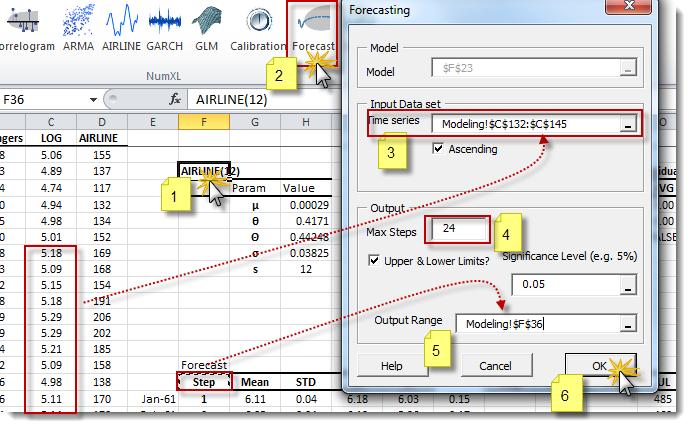Please note that for forecast purposes, the input time series here refers to the latest 13 months, or the observations between Nov 1959 and Dec 1960. The output table is shown below: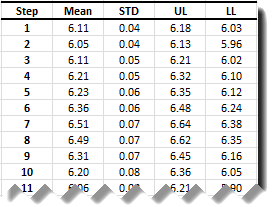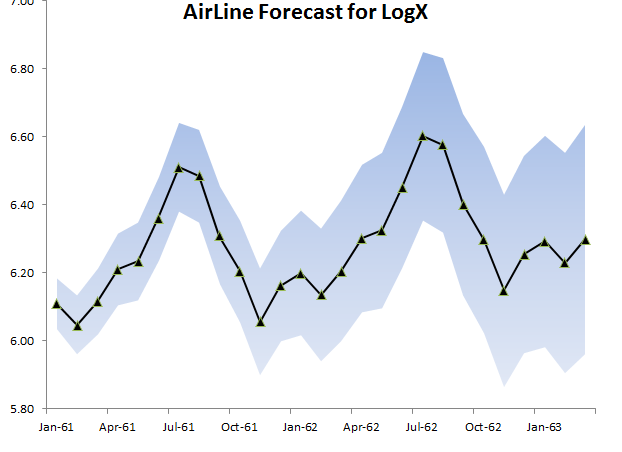To convert back to regular monthly totals, use these equations:

$$UL=e^{UL_{log}}$$

$$LL=e^{LL_{log}}$$

$$\mu=e^{\mu_{log}+\frac{\sigma_{log}^2}{2}}$$